## 14.2.3 Motion Primitives

The discrete-time model of Section 14.2.2 is just one of many possible ways to discretize the space of action trajectories. It will now be considered as a special case of specifying motion primitives. The restriction to constant actions over fixed time intervals may be too restrictive in many applications. Suppose we want to automate the motions of a digital actor for use in a video game or film. Imagine having a database of interesting motion primitives. Such primitives could be extracted, for example, from motion capture data [35,553]. For example, if the actor is designed for kung-fu fighting, then each motion sequence may correspond to a basic move, such a kick or punch. It is unlikely that such motion primitives correspond to constant actions over a fixed time interval. The durations of the motion primitives will usually vary.

Such models can generally be handled by defining a more general kind of discretization. The discrete-time model can be used to formulate a discrete-time state transition equation of the form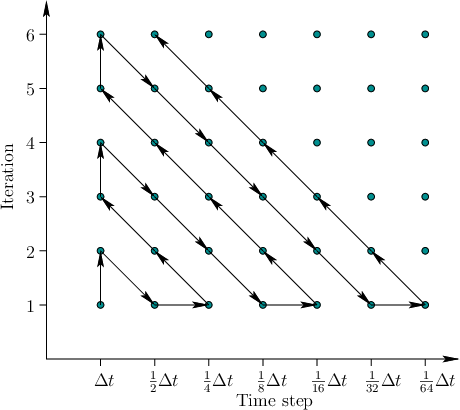(14.12)

in which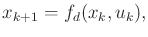,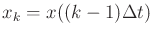, and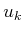is the action in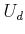that is applied from time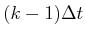to time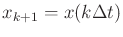. Thus,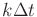is a function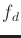that represents an approximation to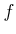, the original state transition function. Every constant action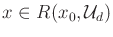applied over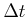can be considered as a motion primitive.

Now generalize the preceding construction to allow more general motion primitives. Let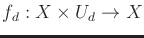denote a motion primitive, which is a function from an interval of time into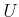. Let the interval of time start at 0 and stop at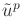, which is a final time that depends on the particular primitive. From any state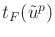, suppose that a set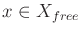of motion primitives is available. The set may even be infinite, in which case some additional sampling must eventually be performed over the space of motion primitives by a local planning method. A state transition equation that operates over discrete stages can be defined as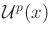(14.13)

in which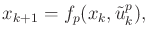is a motion primitive that must be chosen from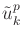. The time discretization model and (14.12) can be considered as a special case in which the motion primitives are all constant over a fixed time interval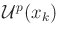. Note that in (14.13) the stage indexdoes not necessarily correspond to time. The indexmerely represents the fact that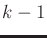motion primitives have been applied so far, and it is time to decide on theth motion primitive. The current time is determined by summing the durations of allprimitives applied so far. If a setof primitives is given for all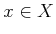, then a reachability graph and its swath can be defined by simple extensions of the discrete-time case. The discrete-time model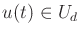can now be interpreted as a special set of motion primitives.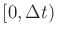For some motion primitives, it may not be possible to immediately sequence them without applying transitional motions. For example, in , two different kinds of motion primitives, called trim trajectories and maneuvers, are defined for autonomous helicopter flight. The trim trajectories correspond to steady motions, and maneuvers correspond to unsteady motions that are needed to make transitions between steady motions. Transitions from one trim trajectory to another are only permitted through the execution of a maneuver. The problem can be nicely modeled as a hybrid system in which each motion primitive represents a mode  (recall hybrid system concepts from Sections 7.3, 8.3.1, and 10.6). The augmented state space is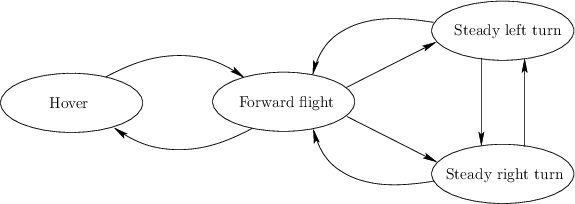, in whichis a set of modes. The transition equation (14.13) can be extended over the augmented state space so that motion primitives can change modes in addition to changing the original state. The possible trajectories for the helicopter follow paths in a graph called the maneuver automaton. An example from  is shown in Figure 14.8. Every edge and every vertex corresponds to a mode in the maneuver automaton. Each edge or vertex actually corresponds to a parameterized family of primitives, from which a particular one is chosen based on the state. A similar state machine is proposed in  for animating humans, and the motion primitives are called behaviors.

Discretizations based on general motion primitives offer great flexibility, and in many cases dramatic performance improvements can be obtained in a sampling-based planning algorithm. The main drawback is that the burden of establishing resolution completeness is increased.

Steven M LaValle 2012-04-20